Category: math

A comment-thread well worth following while on vacation was Algebraic Geometry without Prime Ideals at the Secret Blogging Seminar. Peter Woit became lyric about it :

My nomination for the all-time highest quality discussion ever held in a blog comment section goes to the comments on this posting at Secret Blogging Seminar, where several of the best (relatively)-young algebraic geometers in the business discuss the foundations of the subject and how it should be taught.

I follow far too few comment-sections to make such a definite statement, but found the contributions by James Borger and David Ben-Zvi of exceptional high quality. They made a case for using Grothendieck’s ‘functor of points’ approach in teaching algebraic geometry instead of the ‘usual’ approach via prime spectra and their structure sheaves.

The text below was written on december 15th of last year, but never posted. As far as I recall it was meant to be part two of the ‘Brave New Geometries’-series starting with the Mumford’s treasure map post. Anyway, it may perhaps serve someone unfamiliar with Grothendieck’s functorial approach to make the first few timid steps in that directions.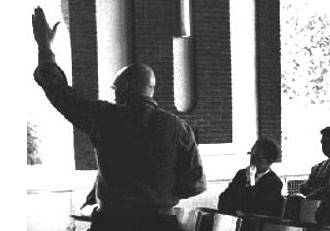Allyn Jackson’s beautiful account of Grothendieck’s life “Comme Appele du Neant, part II” (the first part of the paper can be found here) contains this gem :

“One striking characteristic of Grothendieck’s
mode of thinking is that it seemed to rely so little
on examples. This can be seen in the legend of the
so-called “Grothendieck prime”.

In a mathematical
conversation, someone suggested to Grothendieck
that they should consider a particular prime number.
“You mean an actual number?” Grothendieck
asked. The other person replied, yes, an actual
prime number. Grothendieck suggested, “All right,
take 57.”

But Grothendieck must have known that 57 is not
prime, right? Absolutely not, said David Mumford
of Brown University. “He doesn’t think concretely.””

We have seen before how Mumford’s doodles allow us to depict all ‘points’ of the affine scheme $\mathbf{spec}(\mathbb{Z}[x])$, that is, all prime ideals of the integral polynomial ring $\mathbb{Z}[x]$.
Perhaps not too surprising, in view of the above story, Alexander Grothendieck pushed the view that one should consider all ideals, rather than just the primes. He achieved this by associating the ‘functor of points’ to an affine scheme.

Consider an arbitrary affine integral scheme $X$ with coordinate ring $\mathbb{Z}[X] = \mathbb{Z}[t_1,\ldots,t_n]/(f_1,\ldots,f_k)$, then any ringmorphism
$\phi~:~\mathbb{Z}[t_1,\ldots,t_n]/(f_1,\ldots,f_k) \rightarrow R$
is determined by an n-tuple of elements $~(r_1,\ldots,r_n) = (\phi(t_1),\ldots,\phi(t_n))$ from $R$ which must satisfy the polynomial relations $f_i(r_1,\ldots,r_n)=0$. Thus, Grothendieck argued, one can consider $~(r_1,\ldots,r_n)$ an an ‘$R$-point’ of $X$ and all such tuples form a set $h_X(R)$ called the set of $R$-points of $X$. But then we have a functor

$h_X~:~\mathbf{commutative rings} \rightarrow \mathbf{sets} \qquad R \mapsto h_X(R)=Rings(\mathbb{Z}[t_1,\ldots,t_n]/(f_1,\ldots,f_k),R)$

So, what is this mysterious functor in the special case of interest to us, that is when $X = \mathbf{spec}(\mathbb{Z}[x])$?
Well, in that case there are no relations to be satisfied so any ringmorphism $\mathbb{Z}[x] \rightarrow R$ is fully determined by the image of $x$ which can be any element $r \in R$. That is, $Ring(\mathbb{Z}[x],R) = R$ and therefore Grothendieck’s functor of points
$h_{\mathbf{spec}(\mathbb{Z}[x]}$ is nothing but the forgetful functor.

But, surely the forgetful functor cannot give us interesting extra information on Mumford’s drawing?
Well, have a look at the slightly extended drawing below :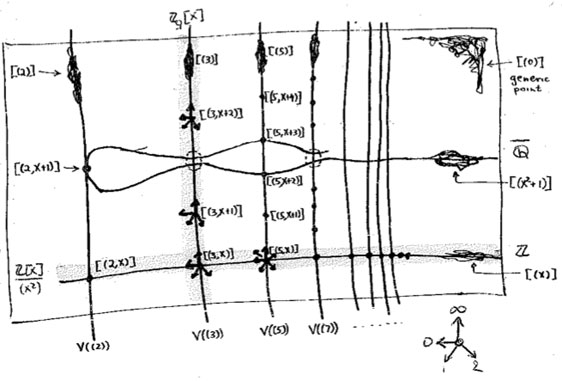What are these ‘smudgy’ lines and ‘spiky’ points? Well, before we come to those let us consider the easier case of identifying the $R$-points in case $R$ is a domain. Then, for any $r \in R$, the inverse image of the zero prime ideal of $R$ under the ringmap $\phi_r~:~\mathbb{Z}[x] \rightarrow R$ must be a prime ideal of $\mathbb{Z}[x]$, that is, something visible in Mumford’s drawing. Let’s consider a few easy cases :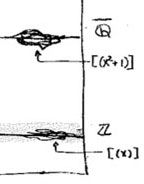For starters, what are the $\mathbb{Z}$-points of $\mathbf{spec}(\mathbb{Z}[x])$? Any natural number $n \in \mathbb{Z}$ determines the surjective ringmorphism $\phi_n~:~\mathbb{Z}[x] \rightarrow \mathbb{Z}$ identifying $\mathbb{Z}$ with the quotient $\mathbb{Z}[x]/(x-n)$, identifying the ‘arithmetic line’ $\mathbf{spec}(\mathbb{Z}) = { (2),(3),(5),\ldots,(p),\ldots, (0) }$ with the horizontal line in $\mathbf{spec}(\mathbb{Z}[x])$ corresponding to the principal ideal $~(x-n)$ (such as the indicated line $~(x)$).

When $\mathbb{Q}$ are the rational numbers, then $\lambda = \frac{m}{n}$ with $m,n$ coprime integers, in which case we have $\phi_{\lambda}^{-1}(0) = (nx-m)$, hence we get again an horizontal line in $\mathbf{spec}(\mathbb{Z}[x])$. For $\overline{\mathbb{Q}}$, the algebraic closure of $\mathbb{Q}$ we have for any $\lambda$ that $\phi_{\lambda}^{-1}(0) = (f(x))$ where $f(x)$ is a minimal integral polynomial for which $\lambda$ is a root.
But what happens when $K = \mathbb{C}$ and $\lambda$ is a trancendental number? Well, in that case the ringmorphism $\phi_{\lambda}~:~\mathbb{Z}[x] \rightarrow \mathbb{C}$ is injective and therefore $\phi_{\lambda}^{-1}(0) = (0)$ so we get the whole arithmetic plane!

In the case of a finite field $\mathbb{F}_{p^n}$ we have seen that there are ‘fat’ points in the arithmetic plane, corresponding to maximal ideals $~(p,f(x))$ (with $f(x)$ a polynomial of degree $n$ which remains irreducible over $\mathbb{F}_p$), having $\mathbb{F}_{p^n}$ as their residue field. But these are not the only $\mathbb{F}_{p^n}$-points. For, take any element $\lambda \in \mathbb{F}_{p^n}$, then the map $\phi_{\lambda}$ takes $\mathbb{Z}[x]$ to the subfield of $\mathbb{F}_{p^n}$ generated by $\lambda$. That is, the $\mathbb{F}_{p^n}$-points of $\mathbf{spec}(\mathbb{Z}[x])$ consists of all fat points with residue field $\mathbb{F}_{p^n}$, together with slightly slimmer points having as their residue field $\mathbb{F}_{p^m}$ where $m$ is a divisor of $n$. In all, there are precisely $p^n$ (that is, the number of elements of $\mathbb{F}_{p^n}$) such points, as could be expected.

Things become quickly more interesting when we consider $R$-points for rings containing nilpotent elements.

Arnold has written a follow-up to the paper mentioned last time called “Polymathematics : is mathematics a single science or a set of arts?” (or here for a (huge) PDF-conversion).

On page 8 of that paper is a nice summary of his 25 trinities :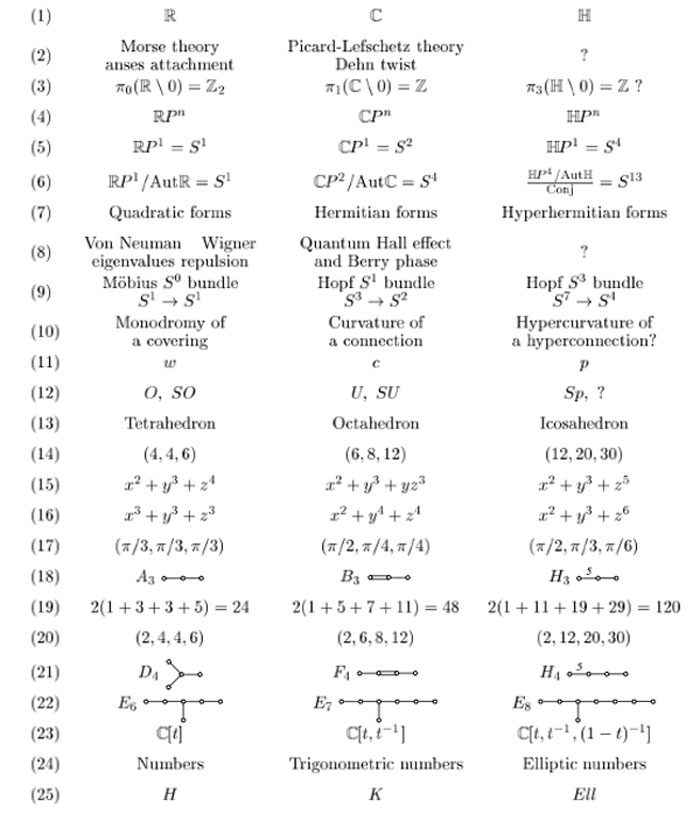I learned of this newer paper from a comment by Frederic Chapoton who maintains a nice webpage dedicated to trinities.

In his list there is one trinity on sporadic groups :where $F_{24}$ is the Fischer simple group of order $2^{21}.3^{16}.5^2.7^3.11.13.17.23.29 = 1255205709190661721292800$, which is the third largest sporadic group (the two larger ones being the Baby Monster and the Monster itself).

I don’t know what the rationale is behind this trinity. But I’d like to recall the (Baby)Monster history as a warning against the trinity-reflex. Sometimes, there is just no way to extend a would be trinity.

The story comes from Mark Ronan’s book Symmetry and the Monster on page 178.

Let’s remind ourselves how we got here. A few years earlier, Fischer has created his ‘transposition’ groups Fi22, Fi23, and Fi24. He had called them M(22), M(23), and M(24), because they were related to Mathieu’s groups M22,M23, and M24, and since he used Fi22 to create his new group of mirror symmetries, he tentatively called it $M^{22}$.
It seemed to appear as a cross-section in something even bigger, and as this larger group was clearly associated with Fi24, he labeled it $M^{24}$. Was there something in between that could be called $M^{23}$?
Fischer visited Cambridge to talk on his new work, and Conway named these three potential groups the Baby Monster, the Middle Monster, and the Super Monster. When it became clear that the Middle Monster didn’t exist, Conway settled on the names Baby Monster and Monster, and this became the standard terminology.

Marcus du Sautoy’s account in Finding Moonshine is slightly different. He tells on page 322 that the Super Monster didn’t exist. Anyone knowing the factual story?

Some mathematical trickery later revealed that the Super Monster was going to be impossible to build: there were certain features that contradicted each other. It was just a mirage, which vanished under closer scrutiny. But the other two were still looking robust. The Middle Monster was rechristened simply the Monster.

And, the inclusion diagram of the sporadic simples tells yet another story.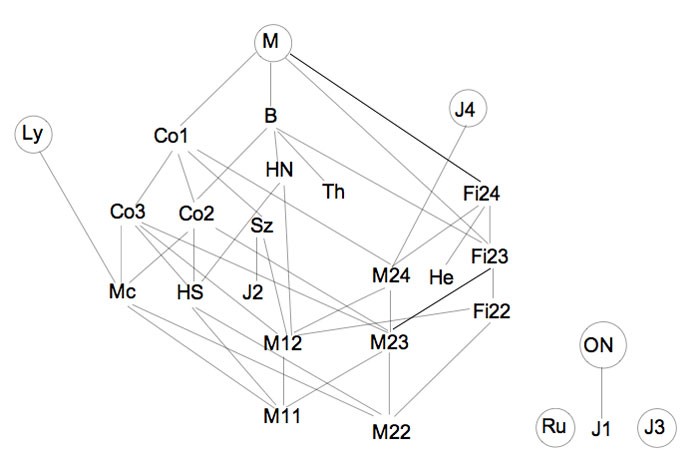Anyhow, this inclusion diagram is helpful in seeing the three generations of the Happy Family (as well as the Pariahs) of the sporadic groups, terminology invented by Robert Griess in his 100+p Inventiones paper on the construction of the Monster (which he liked to call, for obvious reasons, the Friendly Giant denoted by FG).
The happy family appears in Table 1.1. of the introduction.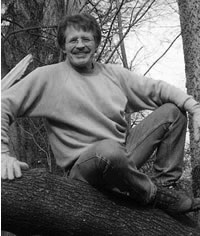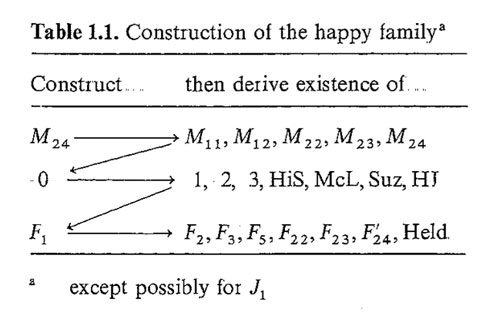It was this picture that made me propose the trinity on the left below in the previous post. I now like to add another trinity on the right, and, the connection between the two is clear.Here $Golay$ denotes the extended binary Golay code of which the Mathieu group $M_{24}$ is the automorphism group. $Leech$ is of course the 24-dimensional Leech lattice of which the automorphism group is a double cover of the Conway group $Co_1$. $Griess$ is the Griess algebra which is a nonassociative 196884-dimensional algebra of which the automorphism group is the Monster.

I am aware of a construction of the Leech lattice involving the quaternions (the icosian construction of chapter 8, section 2.2 of SPLAG). Does anyone know of a construction of the Griess algebra involving octonions???Referring to the triple of exceptional Galois groups $L_2(5),L_2(7),L_2(11)$ and its connection to the Platonic solids I wrote : “It sure seems that surprises often come in triples…”. Briefly I considered replacing triples by trinities, but then, I didnt want to sound too mystic…

David Corfield of the n-category cafe and a dialogue on infinity (and perhaps other blogs I’m unaware of) pointed me to the paper Symplectization, complexification and mathematical trinities by Vladimir I. Arnold. (Update : here is a PDF-conversion of the paper)

The paper is a write-up of the second in a series of three lectures Arnold gave in june 1997 at the meeting in the Fields Institute dedicated to his 60th birthday. The goal of that lecture was to explain some mathematical dreams he had.

The next dream I want to present is an even more fantastic set of theorems and conjectures. Here I also have no theory and actually the ideas form a kind of religion rather than mathematics.
The key observation is that in mathematics one encounters many trinities. I shall present a list of examples. The main dream (or conjecture) is that all these trinities are united by some rectangular “commutative diagrams”.
I mean the existence of some “functorial” constructions connecting different trinities. The knowledge of the existence of these diagrams provides some new conjectures which might turn to be true theorems.

Follows a list of 12 trinities, many taken from Arnold’s field of expertise being differential geometry. I’ll restrict to the more algebraically inclined ones.

1 : “The first trinity everyone knows is”where $\mathbb{H}$ are the Hamiltonian quaternions. The trinity on the left may be natural to differential geometers who see real and complex and hyper-Kaehler manifolds as distinct but related beasts, but I’m willing to bet that most algebraists would settle for the trinity on the right where $\mathbb{O}$ are the octonions.

2 : The next trinity is that of the exceptional Lie algebras E6, E7 and E8.with corresponding Dynkin-Coxeter diagramsArnold has this to say about the apparent ubiquity of Dynkin diagrams in mathematics.

Manin told me once that the reason why we always encounter this list in many different mathematical classifications is its presence in the hardware of our brain (which is thus unable to discover a more complicated scheme).
I still hope there exists a better reason that once should be discovered.

Amen to that. I’m quite hopeful human evolution will overcome the limitations of Manin’s brain…

3 : Next comes the Platonic trinity of the tetrahedron, cube and dodecahedronClearly one can argue against this trinity as follows : a tetrahedron is a bunch of triangles such that there are exactly 3 of them meeting in each vertex, a cube is a bunch of squares, again 3 meeting in every vertex, a dodecahedron is a bunch of pentagons 3 meeting in every vertex… and we can continue the pattern. What should be a bunch a hexagons such that in each vertex exactly 3 of them meet? Well, only one possibility : it must be the hexagonal tiling (on the left below). And in normal Euclidian space we cannot have a bunch of septagons such that three of them meet in every vertex, but in hyperbolic geometry this is still possible and leads to the Klein quartic (on the right). Check out this wonderful post by John Baez for more on this.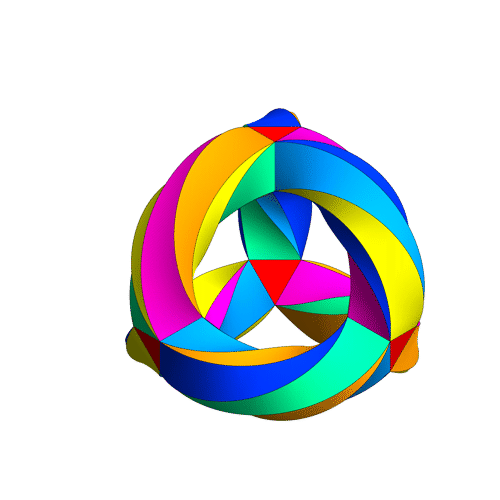4 : The trinity of the rotation symmetry groups of the three Platonicswhere $A_n$ is the alternating group on n letters and $S_n$ is the symmetric group.

Clearly, any rotation of a Platonic solid takes vertices to vertices, edges to edges and faces to faces. For the tetrahedron we can easily see the 4 of the group $A_4$, say the 4 vertices. But what is the 4 of $S_4$ in the case of a cube? Well, a cube has 4 body-diagonals and they are permuted under the rotational symmetries. The most difficult case is to see the $5$ of $A_5$ in the dodecahedron. Well, here’s the solution to this riddle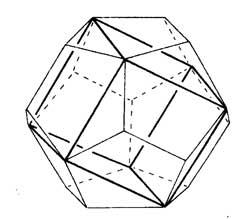there are exactly 5 inscribed cubes in a dodecahedron and they are permuted by the rotations in the same way as $A_5$.

7 : The seventh trinity involves complex polynomials in one variablethe Laurant polynomials and the modular polynomials (that is, rational functions with three poles at 0,1 and $\infty$.

8 : The eight one is another beautyHere ‘numbers’ are the ordinary complex numbers $\mathbb{C}$, the ‘trigonometric numbers’ are the quantum version of those (aka q-numbers) which is a one-parameter deformation and finally, the ‘elliptic numbers’ are a two-dimensional deformation. If you ever encountered a Sklyanin algebra this will sound familiar.

This trinity is based on a paper of Turaev and Frenkel and I must come back to it some time…

The paper has some other nice trinities (such as those among Whitney, Chern and Pontryagin classes) but as I cannot add anything sensible to it, let us include a few more algebraic trinities. The first one attributed by Arnold to John McKay

13 : A trinity parallel to the exceptional Lie algebra one isbetween the 27 straight lines on a cubic surface, the 28 bitangents on a quartic plane curve and the 120 tritangent planes of a canonic sextic curve of genus 4.

14 : The exceptional Galois groupsexplained last time.

15 : The associated curves with these groups as symmetry groups (as in the previous post)where the ? refers to the mysterious genus 70 curve. I’ll check with one of the authors whether there is still an embargo on the content of this paper and if not come back to it in full detail.

16 : The three generations of sporadic groupsDo you have other trinities you’d like to worship?